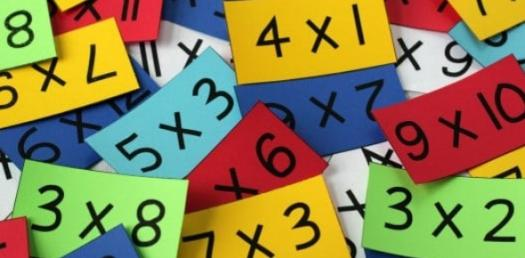# Math Quiz On Basic Multiplication!

Approved & Edited by ProProfs Editorial Team
The editorial team at ProProfs Quizzes consists of a select group of subject experts, trivia writers, and quiz masters who have authored over 10,000 quizzes taken by more than 100 million users. This team includes our in-house seasoned quiz moderators and subject matter experts. Our editorial experts, spread across the world, are rigorously trained using our comprehensive guidelines to ensure that you receive the highest quality quizzes.
| By Krissyxr
K
Krissyxr
Community Contributor
Quizzes Created: 1 | Total Attempts: 1,022
Questions: 10 | Attempts: 1,025SettingsDo you love solving mathematical calculations? Are you smart enough to calculate numbers in mind? Can you multiply big numbers in mind without using a calculator? If yes, then do attempt this quiz to test how fast and sharp you are in solving basic multiplication questions. Read the questions carefully and answer. So, let's try out the quiz. All the best!

• 1.

### 130+120=

• A.

156

• B.

145

• C.

150

• D.

123

• E.

457

C. 150
Explanation
The correct answer is 150 because when you add 130 and 120 together, you get a sum of 250. However, none of the options provided match this sum. Therefore, the answer must be an error or typo, and it is not possible to determine the correct answer based on the given options.

Rate this question:

• 2.

### 20x3=?

• A.

56

• B.

12

• C.

156

• D.

60

D. 60
Explanation
The given question asks for the product of 20 multiplied by 3. Multiplying 20 by 3 gives the result of 60.

Rate this question:

• 3.

### 8x56=?

• A.

448

• B.

123

• C.

357

• D.

678

• E.

398

A. 448
Explanation
The correct answer is 448 because when you multiply 8 by 56, you get the product of 448.

Rate this question:

• 4.

### 24x2=?

• A.

45

• B.

48

• C.

34

• D.

12

• E.

54

B. 48
Explanation
The correct answer is 48 because when you multiply 24 by 2, you get the product of 48.

Rate this question:

• 5.

### 25 x 2 =

• A.

50

• B.

52

• C.

40

• D.

30

A. 50
Explanation
The correct answer is 50 because when you multiply 25 by 2, you are essentially adding 25 to itself 2 times. So, 25 + 25 equals 50.

Rate this question:

• 6.

### 31 x 6 =

• A.

189

• B.

186

• C.

188

• D.

190

B. 186
Explanation
The correct answer is 186. When multiplying 31 by 6, we can use the method of long multiplication. We multiply 6 by the ones digit of 31, which is 1, and get 6. Then we multiply 6 by the tens digit of 31, which is 3, and get 18. We write down the 8 and carry over the 1 to the next column. Finally, we multiply 6 by the hundreds digit of 31, which is 0, and add the carried over 1, resulting in 1. So, the final answer is 186.

Rate this question:

• 7.

### 35 x 22 =

• A.

770

• B.

778

• C.

756

• D.

789

A. 770
Explanation
The correct answer is 770 because when you multiply 35 by 22, you get 770.

Rate this question:

• 8.

### 47 X 25 =

• A.

1175

• B.

1174

• C.

1165

• D.

1135

A. 1175
Explanation
The correct answer is 1175 because when you multiply 47 by 25, the product is equal to 1175.

Rate this question:

• 9.

### 25 X 44 =

• A.

1100

• B.

1121

• C.

1056

• D.

1036

A. 1100
Explanation
The correct answer is 1100 because when you multiply 25 by 44, you get the product of 1100.

Rate this question:

• 10.

### 35 X 45 =

• A.

1564

• B.

1575

• C.

1544

• D.

1574

B. 1575
Explanation
The correct answer is 1575 because when you multiply 35 by 45, you get the product of 1575.

Rate this question:

Related TopicsBack to top